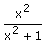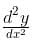JEE  >  Test: Difference of Parametric Functions

# Test: Difference of Parametric Functions

Test Description

## 10 Questions MCQ Test Mathematics (Maths) Class 12 | Test: Difference of Parametric Functions

Test: Difference of Parametric Functions for JEE 2023 is part of Mathematics (Maths) Class 12 preparation. The Test: Difference of Parametric Functions questions and answers have been prepared according to the JEE exam syllabus.The Test: Difference of Parametric Functions MCQs are made for JEE 2023 Exam. Find important definitions, questions, notes, meanings, examples, exercises, MCQs and online tests for Test: Difference of Parametric Functions below.
Solutions of Test: Difference of Parametric Functions questions in English are available as part of our Mathematics (Maths) Class 12 for JEE & Test: Difference of Parametric Functions solutions in Hindi for Mathematics (Maths) Class 12 course. Download more important topics, notes, lectures and mock test series for JEE Exam by signing up for free. Attempt Test: Difference of Parametric Functions | 10 questions in 10 minutes | Mock test for JEE preparation | Free important questions MCQ to study Mathematics (Maths) Class 12 for JEE Exam | Download free PDF with solutions
 1 Crore+ students have signed up on EduRev. Have you?
Test: Difference of Parametric Functions - Question 1

### Differentiate sin2(θ2 + 1) with respect to θ2

Detailed Solution for Test: Difference of Parametric Functions - Question 1

y = sin22+1)
v = θ2
dy/d(v) = dydθ/dvdθ
dy/dthη = sin2(V+1)
= 2sin(V+1)⋅cos(V+1)dv/dθ
= 2sin(θ2+1)cos(θ2+1)
= sin2(θ2+1).

Test: Difference of Parametric Functions - Question 2

### Differentiatewith respect to x2

Test: Difference of Parametric Functions - Question 3

### Find dy/dx if x= a cos θ, y = b sin θ

Test: Difference of Parametric Functions - Question 4

Difference equation in discrete systems is similar to the _____________ in continuous systems.

Detailed Solution for Test: Difference of Parametric Functions - Question 4

Difference equation are the equations used in discrete time systems and difference equations are similar to the differential equation in continuous systems.

Test: Difference of Parametric Functions - Question 5

X = at² and y = 2at are parametric equations of

Detailed Solution for Test: Difference of Parametric Functions - Question 5

Together the equations x = at2 and y = 2at (where t is the parameter) are called the parametric equations of the parabola y2 = 4ax.

Test: Difference of Parametric Functions - Question 6

If x = 4(t + sin t), y = 4(1-cos t), Evaluate dy/dx at t= π/2

Detailed Solution for Test: Difference of Parametric Functions - Question 6

x = a(t+sin t)
⟹dx/dt = a(1+cos t)
And y = a(1−cos t)
⟹dy/dt = a[0−(−sin t)]
=a sin t
Therefore, dy/dx = a sin t/(a(1+cos t))
= 2sin t/2 cos t/2)/(2cos^2 t/2)
=tan(t/2)
At π/2

tan(π/4) = 1

Test: Difference of Parametric Functions - Question 7

Find dy/dx if x = cos3 θ, y = sin3 θ

Detailed Solution for Test: Difference of Parametric Functions - Question 7

x = cos3θ
⇒ dx/dθ = a(3cos2θ)(−sinθ)
= −3sinθcos2θ
y = sin3θ
⇒dy/dθ = a(3sin2θ)(cosθ)
= 3sin2θcosθ
∴dy/dx = (dy/dθ)(dx/dθ)
= (3sin2θcosθ)/(−3asinθcos2θ)
= −sinθ/cosθ
= −tanθ

Test: Difference of Parametric Functions - Question 8

Find; x = 20 (cos t + t sin t) and y = 20 ( sin t - t cos t)

Detailed Solution for Test: Difference of Parametric Functions - Question 8

x = 20(cost + tsint)
differentiate x with respect to t,
dx/dt = 20{d(cost)/dt + d(tsint)/dt]
= 20[-sint + {t. d(sint)/dt + sint.dt/dt}]
= 20[ -sint + tcost + sint]
= -20t.cost
hence, dx/dt = -20t.cost -----(1)

y = 20(sint - tcost)
differentiate y with respect to t,
dy/dt = 20[d(sint)/dt - d(tcost)/dt ]
= 20[cost - {t.d(cost)/dt + cost.dt/dt}]
= 20[cost +tsint -cost]
= 20t.sint
hence, dy/dt = 20t.sint -------(2)

dividing equations (2) by (1),
dy/dx = 20t.sint/20t.cost
dy/dx = tant
now again differentiate with respect to x
d²y/dx² = sec²t. dt/dx ------(3)
now from equation (1),
dx/dt = 20t.cost
so, dt/dx =1/at.cost put it in equation (3),
e.g., d²y/dx² = sec²t. 1/20t.cost
d²y/dx² = sec³t/20t

Test: Difference of Parametric Functions - Question 9

If f (x) = [x sin p x] { where [x] denotes greatest integer function}, then f (x) is

Detailed Solution for Test: Difference of Parametric Functions - Question 9

If −1≤x≤1, then 0≤xsinπx≤1/2
​∴ f(x)=[xsinπx]=0, for −1≤x≤1
If 1<x<1+h, where h is a small positive real number, then
π<πx<π+πh
⇒−1<sinπx<0
⇒−1<xsinπx<0
∴ f(x)=[xsinπx]=−1 in the right neighbourhood of x=1.
Thus, f(x) is constant and equal to zero in [−1,1] and so f(x) is differentiable and hence continuous on (−1,1).

Test: Difference of Parametric Functions - Question 10

Differentiate sin x3 with respect to x3

Detailed Solution for Test: Difference of Parametric Functions - Question 10

Differentiation of sinx3 with respect to x3 is
d(sin(x3)/dx = cosx3

## Mathematics (Maths) Class 12

209 videos|218 docs|139 tests
 Use Code STAYHOME200 and get INR 200 additional OFF Use Coupon Code
Information about Test: Difference of Parametric Functions Page
In this test you can find the Exam questions for Test: Difference of Parametric Functions solved & explained in the simplest way possible. Besides giving Questions and answers for Test: Difference of Parametric Functions, EduRev gives you an ample number of Online tests for practice

## Mathematics (Maths) Class 12

209 videos|218 docs|139 tests